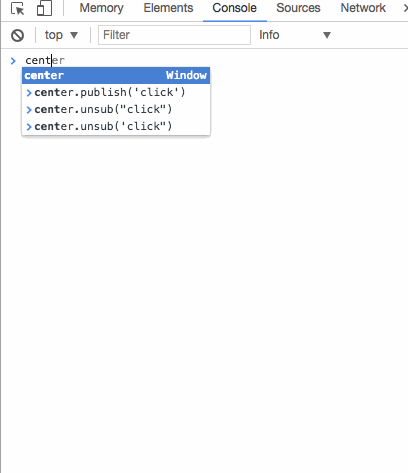``````document.body.addEventListener('click', function() {
}, false)

document.body.click()
``````

``````var center = {}; // 定义信号中心

center.subscribeList = []; // 缓存列表

center.subscribe = function(fn) { // 增加订阅
this.subscribeList.push(fn); // 订阅的消息放入缓存列表
};

center.publish = function() { // 发布消息
for (var i = 0, fn; fn = this.subscribeList[i++];) {
fn.apply(this, arguments);  // 发布时带上参数
}
}

// 下面进行测试，先订阅消息
center.subscribe(function(signal, action) {
console.log('触发信号：' + signal);
console.log('执行动作：' + action);
});

// 发布时输出：
// 触发信号：click
// 执行动作：write something
center.publish('click', 'write something');
``````

``````var center = {}; // 定义中心
center.subscribeList = {};  // 缓存列表

center.subscribe = function(signal, fn) {
if (!this.subscribeList[signal]) {
this.subscribeList[signal] = [];  // 如果没有存放过此类消息才创建一个针对此类消息的缓存列表
}
this.subscribeList[signal].push(fn);
};

center.publish = function() {
var signal = Array.prototype.shift.call(arguments);
var fns = this.subscribeList[signal];

if (!fns || fns.length === 0) {
return false;
}

for (var i = 0, fn; fn = fns[i++];) {
fn.apply(this, arguments);
}
}

center.subscribe('click', function() {
console.log('ok someone just clicked something...');
});

center.subscribe('scroll', function() {
console.log('lol stop scrolling, dude!');
})

center.publish('click');
center.publish('scroll');
``````

``````{
'click': [a, b, c, ...],
'scroll': [d, e, f, ...],
...
...
}
``````

``````center.unsub = function(signal, fn) {
var fns = this.subscribeList[signal];

if (!fns) { // 如果这一类信号没有被人订阅，直接返回
return false;
}

if (!fn) {  // 如果没有传入需要取消的具体回调函数，意味着需要移除所有的对应的回调
fns && (fns.length = 0)
} else {
for (var l = fns.length - 1; l >= 0; l--) { // 反向遍历订阅的回调函数列表
var _fn = fns[l];
if (_fn === fn) {
fns.splice(1, 1); // 删除回调函数
}
}
}
}
````````````var event = {
subscribeList: {},

subscribe: function(signal, fn) {
if (!this.subscribeList[signal]) {
this.subscribeList[signal] = [];
}
this.subscribeList[signal].push(fn);
},

publish: function() {
var signal = Array.prototype.shift.call(arguments);
var fns = this.subscribeList[signal];

if (!fns || fns.length === 0) {
return false;
}

for (var i = 0, fn; fn = fns[i++];) {
fn.apply(this, arguments);
}
},

unsub: function(signal, fn) {
var fns = this.subscribeList[signal];

if (!fns) {
return false;
}

if (!fn) {
fns && (fns.length = 0)
} else {
for (var l = fns.length - 1; l >= 0; l--) {
var _fn = fns[l];
if (_fn === fn) {
fns.splice(1, 1);
}
}
}
},

installEvent: function(obj) {
for (var i in event) {
obj[i] = event[i];
}
}
}
``````